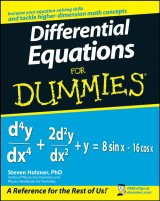# Details## Differential Equations For Dummies

1. Aufl.
 von: Steven Holzner 16,99 € Verlag: Wiley Format: PDF Veröffentl.: 02.09.2008 ISBN/EAN: 9780470395660 Sprache: englisch Anzahl Seiten: 368

DRM-geschütztes eBook, Sie benötigen z.B. Adobe Digital Editions und eine Adobe ID zum Lesen.

## Beschreibungen

#### Titelbeschreibung

<b>The fun and easy way to understand and solve complex equations</b> <p>Many of the fundamental laws of physics, chemistry, biology, and economics can be formulated as differential equations. This plain-English guide explores the many applications of this mathematical tool and shows how differential equations can help us understand the world around us. <i>Differential Equations For Dummies</i> is the perfect companion for a college differential equations course and is an ideal supplemental resource for other calculus classes as well as science and engineering courses. It offers step-by-step techniques, practical tips, numerous exercises, and clear, concise examples to help readers improve their differential equation-solving skills and boost their test scores.</p>

#### Inhaltsverzeichnis

<b>Introduction.</b> <p><b>Part I: Focusing on First Order Differential Equations.</b></p> <p>Chapter 1: Welcome to the World of Differential Equations.</p> <p>Chapter 2: Looking at Linear First Order Differential Equations.</p> <p>Chapter 3: Sorting Out Separable First Order Differential Equations.</p> <p>Chapter 4: Exploring Exact First Order Differential Equations and Euler’s Method.</p> <p><b>Part II: Surveying Second and Higher Order Differential Equations.</b></p> <p>Chapter 5: Examining Second Order Linear Homogeneous Differential Equations.</p> <p>Chapter 6: Studying Second Order Linear Nonhomogeneous Differential Equations.</p> <p>Chapter 7: Handling Higher Order Linear Homogeneous Differential Equations.</p> <p>Chapter 8: Taking On Higher Order Linear Nonhomogeneous Differential Equations.</p> <p><b>Part III: The Power Stuff: Advanced Techniques.</b></p> <p>Chapter 9: Getting Serious with Power Series and Ordinary Points.</p> <p>Chapter 10: Powering through Singular Points.</p> <p>Chapter 11: Working with Laplace Transforms.</p> <p>Chapter 12: Tackling Systems of First Order Linear Differential Equations.</p> <p>Chapter 13: Discovering Three Fail-Proof Numerical Methods.</p> <p><b>Part IV: The Part of Tens.</b></p> <p>Chapter 14: Ten Super-Helpful Online Differential Equation Tutorials.</p> <p>Chapter 15: Ten Really Cool Online Differential Equation Solving Tools.</p> <p><b>Index.</b></p>

#### Autorenportrait

<b>Steven Holzner</b> is an award-winning author of science, math, and technical books. He got his training in differential equations at MIT and at Cornell University, where he got his PhD. He has been on the faculty at both MIT and Cornell University, and has written such bestsellers as <i>Physics For Dummies</i> and <i>Physics Workbook For Dummies.</i>

#### Back cover copy

<b>Power your way through ordinary and singular points</b> <p><b>Understand differential equations through practical tips and examples</b></p> <p>Do differential equations cause you distress? No worries! This friendly guide explains this intimidating subject in plain English, walking you step by step through all the key concepts — from linear and separable first order differential equations to higher order equations, power series, and Laplace transforms. You'll find plenty of examples to increase your problem-solving skills and a variety of helpful definitions and explanations to conquer even the toughest differential equations.</p> <p><b>Discover how to:</b></p> <ul> <li> <p>Classify differential equations</p> </li> <li> <p>Solve with integrating factors</p> </li> <li> <p>Work with coefficients</p> </li> <li> <p>Use handy theorems</p> </li> <li> <p>Have fun with advanced techniques</p> </li> <li> <p>Apply differential equations in real life</p> </li> </ul>

US